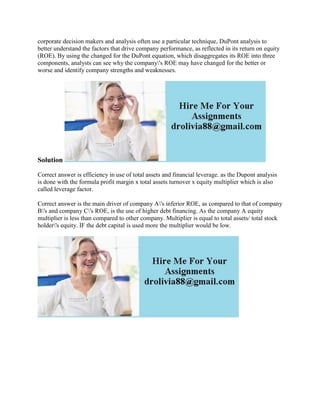Se está descargando tu SlideShare. ×

# corporate decision makers and analysis often use a particular techniqu.docx

Anuncio
Anuncio
Anuncio
Anuncio
Anuncio
Anuncio
Anuncio
Anuncio
Anuncio
Anuncio
AnuncioCargando en…3
×

1 de 1 Anuncio

# corporate decision makers and analysis often use a particular techniqu.docx

corporate decision makers and analysis often use a particular technique, DuPont analysis to better understand the factors that drive company performance, as reflected in its return on equity (ROE). By using the changed for the DuPont equation, which disaggregates its ROE into three components, analysts can see why the company\'s ROE may have changed for the better or worse and identify company strengths and weaknesses.
Solution
Correct answer is efficiency in use of total assets and financial leverage. as the Dupont analysis is done with the formula profit margin x total assets turnover x equity multiplier which is also called leverage factor.
Correct answer is the main driver of company A\'s inferior ROE, as compared to that of company B\'s and company C\'s ROE, is the use of higher debt financing. As the company A equity multiplier is less than compared to other company. Multiplier is equal to total assets/ total stock holder\'s equity. IF the debt capital is used more the multiplier would be low.
.

corporate decision makers and analysis often use a particular technique, DuPont analysis to better understand the factors that drive company performance, as reflected in its return on equity (ROE). By using the changed for the DuPont equation, which disaggregates its ROE into three components, analysts can see why the company\'s ROE may have changed for the better or worse and identify company strengths and weaknesses.
Solution
Correct answer is efficiency in use of total assets and financial leverage. as the Dupont analysis is done with the formula profit margin x total assets turnover x equity multiplier which is also called leverage factor.
Correct answer is the main driver of company A\'s inferior ROE, as compared to that of company B\'s and company C\'s ROE, is the use of higher debt financing. As the company A equity multiplier is less than compared to other company. Multiplier is equal to total assets/ total stock holder\'s equity. IF the debt capital is used more the multiplier would be low.
.

Anuncio
Anuncio

### corporate decision makers and analysis often use a particular techniqu.docx

1. 1. corporate decision makers and analysis often use a particular technique, DuPont analysis to better understand the factors that drive company performance, as reflected in its return on equity (ROE). By using the changed for the DuPont equation, which disaggregates its ROE into three components, analysts can see why the company's ROE may have changed for the better or worse and identify company strengths and weaknesses. Solution Correct answer is efficiency in use of total assets and financial leverage. as the Dupont analysis is done with the formula profit margin x total assets turnover x equity multiplier which is also called leverage factor. Correct answer is the main driver of company A's inferior ROE, as compared to that of company B's and company C's ROE, is the use of higher debt financing. As the company A equity multiplier is less than compared to other company. Multiplier is equal to total assets/ total stock holder's equity. IF the debt capital is used more the multiplier would be low.